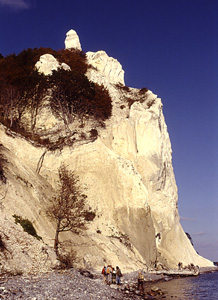STRUCTURAL GEOLOGY EXERCISES with Glaciotectonic Examples James S. Aber, Professor Emeritus Emporia State University, Kansas Exercise Solutions

 Planar strata Primary structure Fold geometry Structure contouring Wulff stereonet Schmidt stereonet I Schmidt stereonet II Rock strength Fault geometry Plate motion Polar wandering Back to exercises

### 2. PLANAR STRATA

1. See map solution (below). First connect points and label: A = lowest at -155, B = intermediate at 95, and C = highest at 145. Locate point D along line AC:

```	elevation B - elevation A     250   distance AD     AD
-------------------------  =  --- = -----------  = ----
elevation C - elevation A     300   distance AC    1200```
AD = 1000, and line BD is the strike = N45E (225°). Draw the dip line AE perpendicular to line BD. Calculate the dip angle:
```	elevation E - elevation A     250
-------------------------  =  ---  =  tan (dip angle).  Dip angle = 14° (NW).
map distance AE               980
```
2. See map solution (below). First determine the elevation of the mineralized vein at point AMG. Draw a dip line perpendicular to line BD to point AMG. Measure the map distance of this dip line, and use a dip angle of 14° to solve:

```	elevation E (95) - elevation AMG
--------------------------------  =  tan 14°
map distance of dip line (550)      ```
Elevation of AMG = -42 m. Subtract elevation of vein at AMG from land surface elevation to determine depth: 158 - (-42) = 200 m.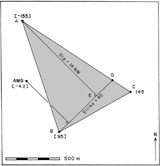Strike-and-dip triangle map

### 3. PRIMARY STRUCTURE

Interpret the regional pattern of paleocurrents as displayed on the rose diagram and the map. Briefly describe the likely depositional environment and paleogeography for the Kiowa and Dakota Formations.

The cross-bedding pattern is bimodal and/or multi-modal with a strong mode toward the southwest, a secondary mode to WNW, and several lesser modes in other directions. The regional pattern is likewise highly variable. This suggests multiple meandering channels on an alluvial plain and/or delta complex.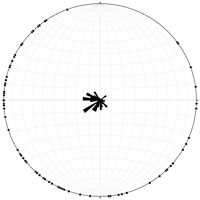Rose diagram for Dakota cross bedding

### 4. FOLD GEOMETRY

1. Apparent dips rounded off to the nearest degree for data points:

A = 38° NE, B = horizontal, C = 51° SW, D = 14° SW, E = 15° NE, F = 55° NE, G = 42° NE, H = 63° NE, and I = 5° SW.

2. See cross section (below). An anticline near the center separates two synclines.

3. Parallel fold geometry can extend no deeper than intersection point E/F, which is 0.5 mile (2640 feet) deep.

4. It can be seen that the folds are plunging because the tilted strata do not have parallel strike measurements. Plunge is to the NW, however the degree of plunge is not great.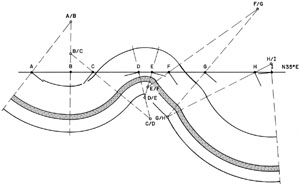Fold cross section

### 5. STRUCTURE CONTOURING

1. See contour map (below) of southeastern Cowley County (adapted from Bayne 1962, plate 5).

2. See structural cross section (profile) on top of the Douglas Group.

3. Map scale: township boundaries are 2.25 inches long on the map, which equals 6 miles, or 31,680 feet, or 380,160 inches. The map scale thus equals 2.25/380,160 or 1:168,960. One mile = 0.375 inches on the map.

The vertical cross-section scale of 1 inch = 200 feet (2400 inches) is 1/2400 or 1:2400. The resulting vertical exaggeration equals 68,960/2400 or approximately 70 times.

4. Regional strike is generally N-S with westward dip of 40 to 70 feet per mile. A line drawn between point 6 (T33S/R7E) and point 4 (T33S/R8E) is nearly perpendicular to contours, and so represents a true dip line.

The two points are approximately 24,640 feet apart and 205 feet different in elevation. Applying the dip-angle formula: 205/24,640 = tan (dip angle); dip angle = 0.5° W, or 44 feet per mile.

5. See contour map of Cowley County. An anticline trends north to northeast through the following points: 7 (T34S/R6E), 6 (T33S/R6E), 1 and 3 (T33S/R7E), 6 and 7 (T32S/R7E). This is the subsurface expression of the Dexter Anticline.

6. Bayne's (1962, plate 5) structure contour map of Cowley County shows a long anticline trending north and northeast across the area. One alternate version is shown by dashed -100 contours near the question mark on the map. This produces a more pronounced closed depression around points 2 and 4 (T33S/R7E).

This variation is perhaps the simplest and the one that many students will give; it does not significantly alter the overall structural pattern, as an anticline is still evident immediately to the west. Bayne's map and this first variation are preferred, because they conform with the surface expression of the Dexter Anticline.

7. A second, more radical, variation involves a trough or syncline plunging westward from points 2 and 4 (T33S/R7E) between point 1 (T33S/R7E) and points 5 and 6 (T33S/R6E), as indicated by dashed -100 and -150 contours near the center of the map. Yet another variation shows a trough extending from the low points 2 and 4 (T33S/R7E) toward the southwest between point 6 (T33S/R6E) and point 7 (T34S/R6E).

These variations do represent significantly different structural patterns, but they are equally correct based on the available subsurface data. Still other contour configur-ations and structures are possible in the central portion of the map area. The instructor should examine each version carefully to ascertain its correctness based on the data.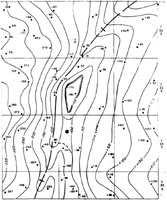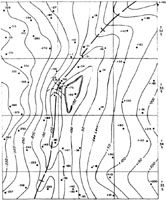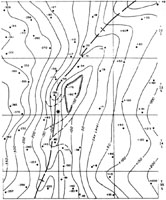Cowley County structure contour map versions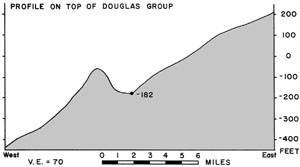Cowley County structure profile

### 6. EQUAL-ANGULAR STEREONET

1. This problem is like example C (see stereonet plot). The intersection of the two fold limbs is a horizontal line trending 104, same as the strike of the two limbs. Axis plots as two "half points" at 104 and 284°.

2. See stereonet plot. The Whitemud and lower Ravenscrag fold axes are subparallel, and they do correspond to the local ESE trend of the Dirt Hills.

3. 24°.

4. Local ice movement came from the NNE toward SSW.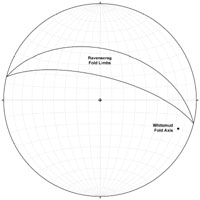Dirt Hills folds

### 7. EQUAL-AREA STEREONET I

1. See stereonet plot, site 3.

2. Parallel fabric: largest cluster of data shows slight plunge toward ENE with small cluster in opposite direction. Secondary cluster shows slight plunge at right angle (SSE).

3. Ice movment from about N70E is indicated.

4. See stereonet plot, site 1.

5. Fabric appears mixed or ambiguous. Many pebbles have greater plunge angles.

6. No, the two fabrics are not similar. Most pebbles in 3 have plunge <25°; whereas many pebbles in 1 have plunge angles >30° with several >70°.

7. See stereonet plot, site 1. About 2/3s of pebble axes plunge in directions between 0-150° and 330-360°, in other words northeast of the thrust plane strike line. A majority of these plunge below (steeper than) the thrust plane.

8. At the time of thrusting and diapiric intrusion of the lower Independence till, the till fabric was reoriented or tilted into a more steeply plunging position.

9. Ice movement from northeast to southwest is indicated.

10. Upper Independence till = Dakota ice lobe; lower Independence till = Minnesota ice lobe.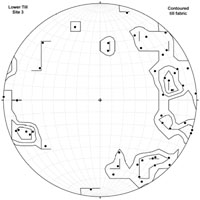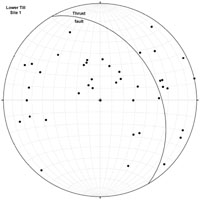Stereonet Plots – Lower Independence till

### 8. EQUAL-AREA STEREONET II

1. See stereonet plot. A constructed fold axis (3) plunging slightly to the WNW is indicated (292/16).

2. Ice movement from NNE toward SSW is indicated.

3. Swedish ice advance(s) from the northeast seems more likely to have folded bedrock at Ertebřlle Hoved than a northerly advance.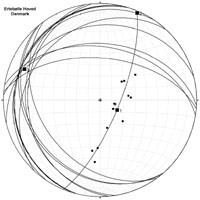Ertebřlle Hoved constructed fold

### 9. ROCK STRENGTH

1. See Mohr diagram (below). Draw large diagrams, as a large diagram can be read more accurately than can a small one. ő1 = 1200 bars, ő3 = 1 atmosphere (1 bar). Center of Mohr circle = 600.5 and radius = 599.5 bars.

2. See Mohr diagram. As ř = 36, then Đ = 27 and 2Đ = 54°. To = app. 310 bars.

3. n = 248 bars and T = 485. Graphical results should be within 5 bars of each.

4. 300 x 0.09 = 27 kg/cm2 (27 bars).

5. See diagram. n = 27 bars in both cases. T = 1 for thawed bed, and T = 10 for frozen bed. Neither type of glacier bed comes anywhere close to the stresses sufficient to fracture intact Irondequoit Limestone.

6. From question 5, it is clear that ice could not fracture intact Irondequoit Limestone. However, pre-existing discontinuities, such as joints, bedding planes, and shale interbeds, were considerably weaker than solid limestone. Thrusting occurred along such discontinuities.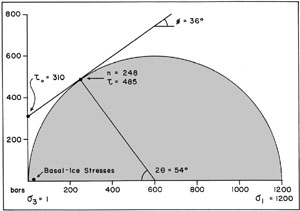Mohr diagram – Irondequoit Limestone

### 10. FAULT GEOMETRY

1. See stereonet plot (below).

2. Yes. All fold axes trend NW or SE with low-angle plunges. Likewise all faults strike NW-SE.

3. Ice movement from NE is indicated. Data cluster in two groups: (1) trend/strike = 100 to 140°, (2) trend/ strike = 160-165°, with no measurements falling in the 140° to 160° range. Corresponds to ice pushing from: (1) about N35E and (2) about N75E.

4. Chalk bodies were derived from the northeast, most likely from what is now the seafloor area of Fakse Bugt.

5. Phase 1 relates to the initial Swedish advance coming from the northeast; phase 2 was created by a Swedish readvance from the ENE.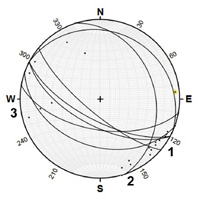Hvideklint folds and faults

### 11. GEOMETRY OF PLATE MOTION

1. To calculate the average linear velocity of seafloor spreading between the Iceland hot spot (point 1) and the Faeroes (point 2), the linear distance between the two along a great circle is determined using the haversine function:

```	havD  =  (cos65 x cos62 x hav10) + hav(65 - 62).  D  =  5.4°.
```
5.4° of a great circle = 5.4 x 111 km = 599.4 (app. 600) km. Average linear velocity equals length divided by time:

```	V  =  600 km/60 million years
V  =  10 km/million years or 1.0 cm per year.
```
2. Seafloor spreading between Iceland and the Faeroes is one half of total seafloor spreading on either side of the Mid-Atlantic ridge. Thus, the average linear velocity of sea-floor spreading between Greenland and the Faeroes is double or 2.0 cm per year.

3. First the colatitude (Cr) of Iceland (point 1) relative to the pole of rotation (point 2) must be calculated using the haversine function:

```	havCr  =  (cos65 x cos78 x hav119) + hav(65 - 78)  [remember: Haversine values are always positive]
Cr  =  32.5°.```
Using V of 2.0 cm per year obtained in question 2 and Cr = 32.5, solve the velocity equation for AmEu:

```	 2.0  =  11.1 AmEu x sin32.5
AmEu  =  0.18018/sin32.5
AmEu  =  0.335 or app. 1/3° per million years. ```
4. First calculate the colatitude of the Azores (point 1) relative to the pole of rotation (point 2) using the haversine function:

`	havCr  =  (cos29 x cos78 x hav132) + hav(29 - 78).  Cr  =  69.4°.`
Now solve for V using AmEu of 0.335° per million years and Cr = 69.4°:

```	V  =  11.1(0.366) x sin69.4
V  =  3.48 (app. 3.5) cm per year.
```
5. Seafloor spreading in vicinity of the Azores during the past 60 million years is: 3.5 cm/year x 60 million years = app. 2100 km.

### 12. POLAR WANDERING

1. Paleolatitude and paleocolatitude (PL and PC) for each rock and the latitude and longitude (Lp and Mp) for each paleopole are:

Site PL PC Lp Mp
1. 47 43 77 200
2. 42 48 71 165
3. 6 84 -40 290 (110)
4. -3 -87 -40 314 (134)
5. 10 80 -34 319 (139)
6. 14 76 -19 332 (152)

2. See polar projection plot (below). Paleopoles 3-6 are located in the southern hemisphere (negative Lp). To plot equivalent poles for northern hemisphere: drop negative signs from Lp and subtract 180 from Mp (values shown in parentheses above).

3. Paleolatitudes for Paleozoic rocks (3-6) are low. North America was located astride the equator and turned on its side, so that what is now western North America faced northward. Thus, the Paleozoic paleopoles appear to be located west of Denver on this plot. Since the Paleozoic, North America has gradually rotated counterclockwise (as viewed from above) and shifted northward, as shown by the Cretaceous and Eocene paleopoles (1-2).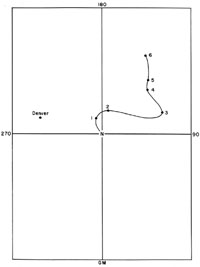Polar wandering chart